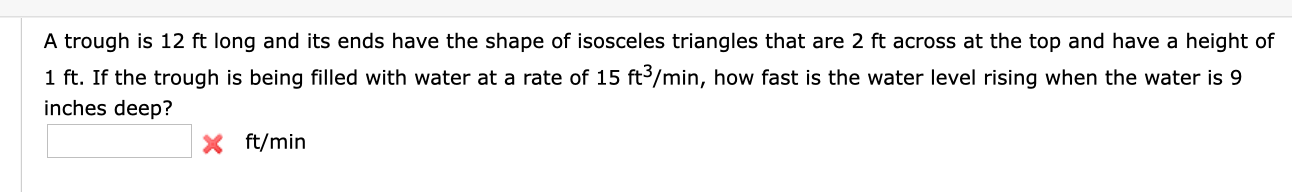# A trough is 12 ft long and its ends have the shape of isosceles triangles that are 2 ft across at the top and have a height of1 ft. If the trough is being filled with water at a rate of 15 ft3/min, how fast is the water level rising when the water is 9inches deep?X ft/min

Questionhelp_outlineImage TranscriptioncloseA trough is 12 ft long and its ends have the shape of isosceles triangles that are 2 ft across at the top and have a height of 1 ft. If the trough is being filled with water at a rate of 15 ft3/min, how fast is the water level rising when the water is 9 inches deep? X ft/min fullscreen
check_circleExpert Solution
Step 1

Let the volume of the water in the trough at time be V. The rate of change of V is,

Step 2

Let h be the depth of water in the trough, which is equal to the height of the triangle which the water makes at the end of the trough.

Step 3

The following equation can be formed which...

### Want to see the full answer?

See Solution

#### Want to see this answer and more?

Solutions are written by subject experts who are available 24/7. Questions are typically answered within 1 hour*

See Solution
*Response times may vary by subject and question
Tagged in

### Calculus